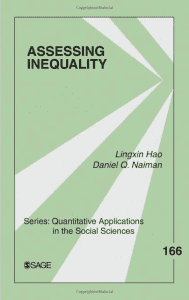# Assessing InequalityProviding basic foundations for measuring inequality from the perspective of distributional properties

This monograph reviews a set of widely used summary inequality measures, and the lesser known relative distribution method provides the basic rationale behind each measure and discusses their interconnections. It also introduces model-based decomposition of inequality over time using quantile regression. This approach enables researchers to estimate two different contributions to changes in inequality between two time points.

Key Features

• Clear statistical explanations provide fundamental statistical basis for understanding the new modeling framework
• Straightforward empirical examples reinforce statistical knowledge and ready-to-use procedures
• Multiple approaches to assessing inequality are introduced by starting with the basic distributional property and providing connections among approaches